# SBI Clerk Mains Reasoning Questions 2019 (Day-05)

Dear Aspirants, Our IBPS Guide team is providing a new series of Reasoning Questions for SBI Clerk /IDBI and South Indian Bank Mains 2019 so the aspirants can practice it on a daily basis. These questions are framed by our skilled experts after understanding your needs thoroughly. Aspirants can practice these new series questions daily to familiarize with the exact exam pattern and make your preparation effective.

Check here for SBI Clerk Mains Mock Test 2019

### Click Here to Subscribe Crack High Level Puzzles & Seating Arrangement Questions PDF 2019 Plan

[WpProQuiz 6750]

Directions (1-5): Study the following information carefully and answer the questions given below.

Eight persons A, B, C, D, G, H, J, and K are sitting around a circular table and all of them are facing inside. Each of them has different ages i.e. 25, 27, 29, 31, 33, 35, 37, and 39 but not necessarily in the same order.

A sits second to the right of G, who is 39 years old. There are two persons sit between A and H. J sits to an immediate left of the one who sits third to the right of C. K’s age is a perfect square. The ages of A, H and C are prime numbers. J is younger than B. The one, whose age is 35, sits second to the left of K. B is not an immediate neighbour of A. A’s age is neither 31 nor 37. K is an immediate neighbour of neither H nor G. H’ age is not 37. C is not an immediate neighbour of G. B’s age is not 35. B does not sit an immediate right of J.

1) Who sits second to the left of the one who sits third to the right of J?

a) G

b) D

c) H

d) A

e) None of these

2) What is the age of the person who sits second to the left of J?

a) 33

b) 37

c) 35

d) 39

e) None of these

3) How many persons sit between G and the one whose age is 33 when counted from the right of G?

a) One

b) Two

c) Three

d) Four

e) None of these

4) What is the position of D with respect to the one whose age is 37?

a) Immediate right

b) Immediate left

c) Third to the left

d) Third to the right

e) None of these

5) If D is related to 25 and A is related to 37; then in the same way which of the following age is C related to?

a) 27

b) 35

c) 29

d) 25

e) None of these

Direction (6-10): In these questions, relationship between different elements is shown in the statements. These statements are followed by two conclusions.

a) If conclusion I and conclusion II follows

b) If Conclusion I follow

c) If Either Conclusion I or Conclusion II follows

d) If Neither Conclusion I nor Conclusion II follows

e) If Conclusion II follows

X \$ Y means P is either greater than or equal to Q

X # Y means P is neither less than nor equal to Q

X @ Y means P is neither greater than nor equal to Q

X & Y means P is equal to Q

X % Y means P is not greater than Q

6)  Statements

A % B # C @ D;    F & C \$ E % G

Conclusions

I) B @ F

II) E @ D

7) Statements

J & K # L \$ M;       L @ N % O & P

Conclusions

I) M # O

II) K @ P

8) Statements

T % U @ V & W;    V \$ X % Y @ Z

Conclusions

I) T @ Y

II) Y % T

9) Statements

F & G @ H % I;     J \$ H @ K # L

Conclusions

I) J # F

II) K # G

10) Statements

P @ Q & R \$ S;      V \$ R % T # U

Conclusions

I) T # P

II) S \$ V

Directions (1-5):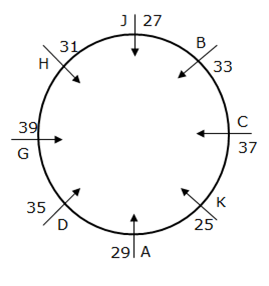A sits second to the right of G, who is 39 years old. There are two persons sit between A and H.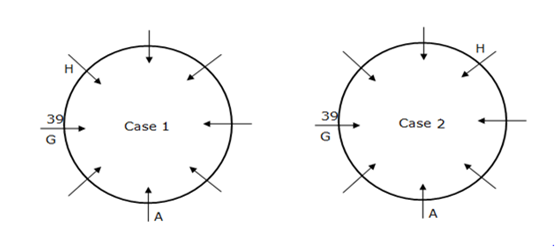K’s age is a perfect square. The one, whose age is 35, sits second to the left of K. K is an immediate neighbour of neither H nor G. The one whose age is 35 sits second to the left of K.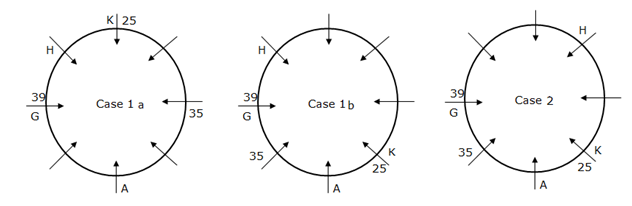J sits an immediate left of the one who sits third to the right of C. C is not an immediate neighbour of G.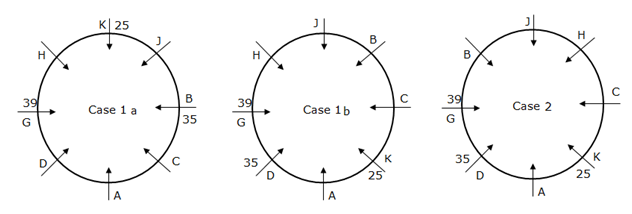The ages of A, H and C are prime numbers. B’s age is not 35. B does not sit an immediate left of J. J is younger than B. B is not an immediate neighbour of A. A’s age is neither 31 nor 37. H’ age is not 37.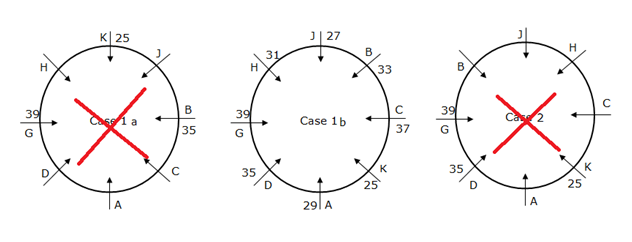Except than prime number ages are 33 and 27, J is younger than B. J is 27 and then B is 33.

In case 1 a, B’s age are not 35, this condition is not satisfied, so rejected.

In case 2, B does not sit an immediate left of J, this condition is not satisfied, so rejected.

So the final solution is Case 1 b.

Direction (6-10) :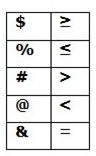I) B < F (B>C=F) –> False

II) E < D (E ≤ C < D) –> TrueI) M > O (M ≤ L < N ≤ O) –> False

II) K < P (K > L < N ≤ O ≥ P) –> FalseI) T < Y (T ≤ U < V ≥ X ≤ Y) –> False

II) Y ≤ T (T ≤ U < V ≥ X ≤ Y) –> False

The above conclusions satisfy Either Or case.I) J > F (J ≥ H > G = F) –> True

II) K > G (K > H > G) –> True